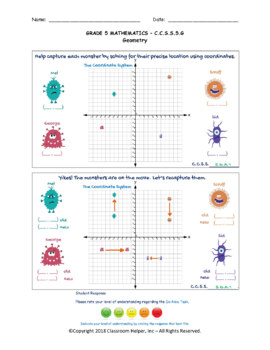# 5th Grade Math Bundle5th
Subjects
Standards
Resource Type
Formats Included
• Zip
•Activity
Pages
59 pages
\$35.00
Bundle
List Price:
\$55.00
You Save:
\$20.00
\$35.00
Bundle
List Price:
\$55.00
You Save:
\$20.00
Easel Activities Included
Some resources in this bundle include ready-to-use interactive activities that students can complete on any device. Easel by TpT is free to use! Learn more.

### Description

Grade 5 Math Do-Now worksheets for Grade 5 mathematics includes arithmetic, number sense, fractions, measurements and data, and geometry.

Arithmetic includes multiplication using area models and standard procedure and division using area models and standard procedure.

Number Sense includes operations with base 10 and place value.

Fractions include equivalent fractions, adding fractions, subtracting fractions, multiplying fractions, and dividing fractions.

Measurements and Data include measurements with real-world applications, converting measurements, volume, real-world data, and the coordinate system.

Geometry includes classifying and identifying two-dimensional shapes, angles, lines, and quadrilaterals.

Total Pages
59 pages
Included
Teaching Duration
N/A
Report this resource to TpT
Reported resources will be reviewed by our team. Report this resource to let us know if this resource violates TpT’s content guidelines.

### Standards

to see state-specific standards (only available in the US).
Use parentheses, brackets, or braces in numerical expressions, and evaluate expressions with these symbols.
Write simple expressions that record calculations with numbers, and interpret numerical expressions without evaluating them. For example, express the calculation “add 8 and 7, then multiply by 2” as 2 × (8 + 7). Recognize that 3 × (18932 + 921) is three times as large as 18932 + 921, without having to calculate the indicated sum or product.
Generate two numerical patterns using two given rules. Identify apparent relationships between corresponding terms. Form ordered pairs consisting of corresponding terms from the two patterns, and graph the ordered pairs on a coordinate plane. For example, given the rule “Add 3” and the starting number 0, and given the rule “Add 6” and the starting number 0, generate terms in the resulting sequences, and observe that the terms in one sequence are twice the corresponding terms in the other sequence. Explain informally why this is so.
Add and subtract fractions with unlike denominators (including mixed numbers) by replacing given fractions with equivalent fractions in such a way as to produce an equivalent sum or difference of fractions with like denominators. For example, 2/3 + 5/4 = 8/12 + 15/12 = 23/12. (In general, 𝘢/𝘣 + 𝘤/𝘥 = (𝘢𝘥 + 𝘣𝘤)/𝘣𝘥.)
Solve word problems involving addition and subtraction of fractions referring to the same whole, including cases of unlike denominators, e.g., by using visual fraction models or equations to represent the problem. Use benchmark fractions and number sense of fractions to estimate mentally and assess the reasonableness of answers. For example, recognize an incorrect result 2/5 + 1/2 = 3/7, by observing that 3/7 < 1/2.

### Questions & Answers

Teachers Pay Teachers is an online marketplace where teachers buy and sell original educational materials.